### Home > PC > Chapter 4 > Lesson 4.1.3 > Problem4-41

4-41.
1. Find the exact values of the following trig expressions. Homework Help ✎

1. cos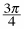2. sin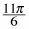3. sin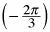4. tan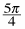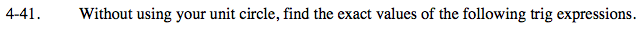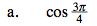1. This is the 2nd quadrant.
2. Cosine is the x-value.
3. Cosine is negative in the 2nd quadrant.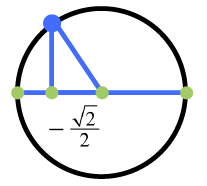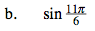1. Sine is y-value.
2. What are the side lengths?
3. Is it positive or negative?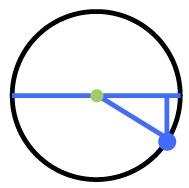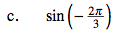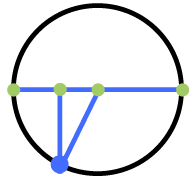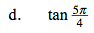$1.\text{ Be sure to take the ratio of }\frac{y-\text{side}}{x-\text{side}}.$